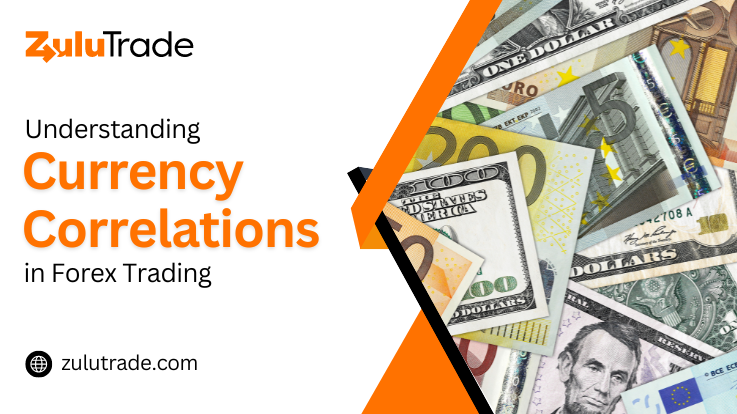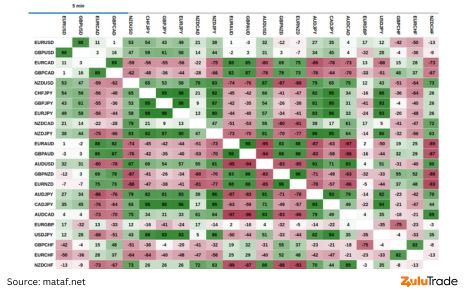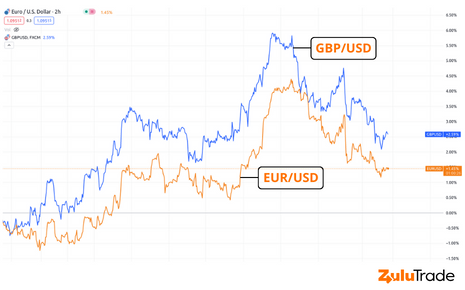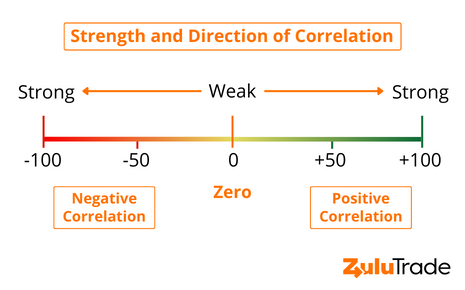# Understanding Currency Correlations in Forex TradingWe see you, dear reader. We know you’re here because the world of Forex trading has piqued your interest and you’re keen on understanding one of its crucial aspects – currency correlations in forex. It can seem like a challenging concept, but don’t worry, you’re not alone in wanting to decipher it.

But what exactly are currency correlations? How can they make a difference to your trading strategies? Will understanding this concept truly give you an edge? If these questions are swirling in your mind, then you’ve come to the right place. You’ll also learn how to read currency correlation tables, use currency correlation tools, and apply this knowledge in your trading strategies.

## What is Currency Correlations in Forex Trading?

Currency Correlation is a statistical measure of the relationship between the movements of two currency pairs, as determined by correlation. If two currencies have a positive correlation, they move in the same direction. If they have a negative correlation, they move in opposite directions. A currency correlation can be strong or weak, and it’s often measured using a currency correlation coefficient.

But why does this matter to a Forex trader? Understanding this can help predict market movements and enhance trading strategies, allowing you to avoid risks and seize opportunities.

## Analyzing Currency Correlation: The Basics

Analyzing currency correlations is all about numbers, but don’t let that scare you off. The currency correlation coefficient is a number between -1 and 1. When two currencies have a correlation of 1, they move perfectly in sync with each other. A correlation of -1 means they move in completely opposite directions. A correlation near 0 means there’s no significant relationship in their movements.

You can calculate these correlations yourself using a currency correlation calculator or use a premade currency correlation matrix available on many Forex trading platforms.

## How to Read Currency Correlation Tables?

Currency correlation tables are valuable tools to visualize the relationship between different currency pairs. They look a lot like a multiplication table, with different currencies listed on both the X and Y axis. The intersection between two currencies will tell you their correlation.## Forex Correlation Pairs and Strategies

There are many forex correlation pairs that traders keep an eye on due to their strong currency correlation. For instance, the EUR/USD and GBP/USD pairs have historically shown a strong positive correlation.

Using these pairs, traders can develop forex trading strategies. For example, if you believe the EUR/USD pair will rise, and the GBP/USD pair has a high positive correlation with it, you could potentially make a profit by buying both pairs.## How to Calculate the Correlation Coefficient?

After understanding what currency correlations are and how they affect your trading, you might be wondering how you can calculate the correlation coefficient yourself. The correlation coefficient is a statistical measure, but don’t let that intimidate you. It’s simpler than it seems, and here’s how you can do it:

First, you need historical price data for the two currency pairs you are interested in. You can easily download this information from your trading platform or other financial data providers.### Calculate Returns:

You can’t directly calculate the correlation between prices; it’s done on returns. To calculate returns, you need to find the percentage change in price from one period to the next. The formula is ((Price at Time 2 – Price at Time 1) / Price at Time 1) * 100%.

### Calculate Average Returns:

Add up all the returns you calculated in step 1 for each currency pair and divide by the number of periods to find the average return.

### Calculate Variance and Standard Deviation:

Variance is a measure of how spread out the returns are from their average. To calculate variance, subtract the average return from each individual return, square the result, and then average these squared results. The standard deviation is simply the square root of the variance.

### Calculate Covariance:

Covariance measures how much two variables move together. To calculate covariance, subtract the average return of one pair from each individual return of that pair, and do the same for the other pair. Multiply these results together, add them all up, and then divide by the number of periods.

### Calculate Correlation Coefficient:

Finally, to calculate the correlation coefficient, divide the covariance by the product of the standard deviations of each currency pair.

This process, while seemingly complex, provides you with the correlation coefficient, a crucial tool for your Forex trading. However, remember that there are also many online tools and calculators available that can do these calculations for you in an instant.

### Using Forex Correlation Tools

There are many tools out there designed to assist traders in understanding and utilizing currency correlations. These include currency correlation calculators, correlation matrixes, and even real-time tracking tools that can alert you to significant changes in currency correlations.

By making use of these tools, you can take your forex trading to the next level, understanding the markets on a deeper level and making more informed trading decisions.

### Understanding Non-Correlated Forex Pairs

As we navigate further into the world of currency correlations, it’s essential to understand what non-correlated Forex pairs mean. Simply put, non-correlated Forex pairs are currency pairs that do not have a significant relationship in their movements. In other words, the changes in one currency pair’s exchange rate won’t give you any meaningful information about the changes in another non-correlated pair’s exchange rate.

This non-correlation is typically represented by a correlation coefficient near zero. If you recall, the correlation coefficient is a measure between -1 and 1, with 1 representing a perfect positive correlation, -1 a perfect negative correlation, and 0 indicating no correlation.

Now, you might wonder, how are non-correlated Forex pairs useful in trading strategies? Here’s the deal: they offer excellent opportunities for diversification. By investing in non-correlated pairs, you can spread the risk associated with market fluctuations. If one currency pair takes a nosedive, your entire portfolio doesn’t have to suffer as your other non-correlated investments might be performing better.

Understanding and effectively using non-correlated Forex pairs can, therefore, be a savvy move to mitigate risks and enhance the stability of your Forex trading portfolio. It’s a little like not putting all your eggs in one basket, a tried-and-true wisdom that holds just as true in the world of Forex trading.

### Wrapping Up

Understanding currency correlations in forex trading may seem daunting at first, but with some practice and the right tools, it becomes an integral part of your trading strategy. As you delve into this topic, remember to use the information you glean not just to predict market movements, but also to diversify your trades and manage risks. It’s not always about finding the perfect pair, but understanding how they all move together in the grand dance of Forex trading.

Posted

in

by

Tags: